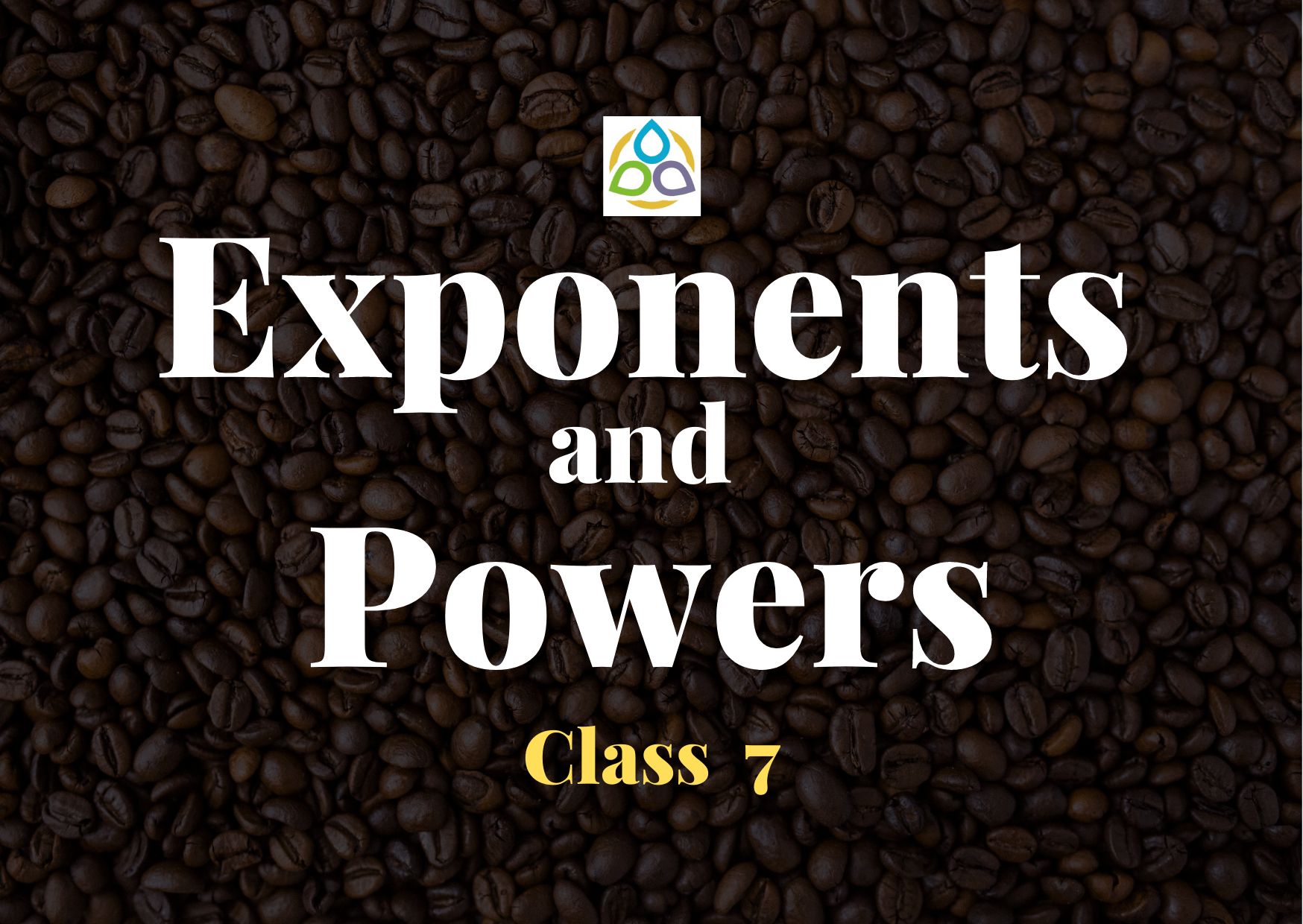# Exponents and Powers

30. What is the value of (91 + 11) × (51 – 41)?

• (A) 10
• (B) 9
• (C) 5
• (D) 4

29. Which among the following is true?

• (A) (52) × 3 = (52)3
• (B) (52) × 3 > (52)3
• (C) (52) × 3 < (52)3
• (D) None of these

28. Which of the following is the correct representation of (–4m)3?

• (A) (–4)3 × (m)3
• (B) 43 × m3
• (C) (–4)3 × m
• (D) 43 × m

27. What is the simplified value of (x7y3/x5y)?

• (A) x2y
• (B) (xy)2
• (C) (xy)3
• (D) xy

26. Which of the following is true (10 + 10)10?

• (A) 1010 + 1010
• (B) 1010 + 100
• (C) 1010 + 101
• (D) None of these

25. Which of the following is true?

• (A) x0 × x0 = x0 ÷ x0
• (B) x0 × x0 = x0 + x0
• (C) x0 × x0 = x0x0
• (D) x0 × x0 = x0 + x0

24. Which of the following is true?

• (A) 34 = 43
• (B) 34 > 43
• (C) 34 < 43
• (D) 34 = 43 × 3

23. Which of the following is true?

• (A) (–3)4 = 12
• (B) (–3)4 > 12
• (C) (–3)4 < 12
• (D) (–3)4 = –12

22. The value of the expression 4 × 105 + 3 × 104 + 2 × 103 + 1 × 100 is

• (A) 432001
• (B) 432010
• (C) 43201
• (D) 430201

21. Which among the following is the correct value of 8020509?

• (A) 8 × 106 + 2 × 104 + 5 × 102 + 9 × 100
• (B) 8 × 106 + 2 × 104 + 5 × 102 + 9 × 100
• (C) 8 × 105 + 2 × 104 + 5 × 102 + 9 × 100
• (D) 8 × 107 + 2 × 104 + 5 × 103 + 9 × 100

20. Which among the following is the correct value of 600060?

• (A) 6 × 105 + 6 × 105 + 6 × 102 + 6 × 102 + 1 × 100
• (B) 6 × 105 + 6 × 102
• (C) 6 × 105 + 6 × 105 + 6 × 102 + 6 × 10 + 0 × 100
• (D) 6 × 105 + 6 × 10

19. Which among the following is the exponential notation for 648?

• (A) 23 × 33
• (B) 23 × 34
• (C) 24 × 34
• (D) 24 × 35

18. The simplified value of [(−2)3 × (−2)4] ÷ (−2)7 is

• (A) 1
• (B) −1
• (C) −2
• (D) 2

17. If a6 × a5 × a0 = ap, the value of p is

• (A) 12
• (B) 11
• (C) 0
• (D) 30

16. If 5x × 53 = 56, the value of x is

• (A) –1
• (B) 0
• (C) 3
• (D) 1